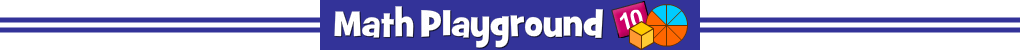Math and strategy team up in Math Surpass Fractions.
Solve the problem and find the answer on the game board.
There may be more than one of the same answer.
That's the strategy part. Which answer should you choose?
The goal is to claim as much of the board as possible.
Create an opponent "sandwich" to earn even more points.
Math Surpass Fractions - Learning Connections
Essential Skills
Mental Math - find a fraction of a number

Common Core Connection for Grade 4
Apply and extend previous understandings of multiplication to multiply a fraction by a whole number.
Understand a fraction a/b as a multiple of 1/b.
Use this understanding to multiply a fraction by a whole number.
More Math Games to Play